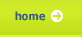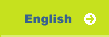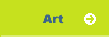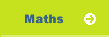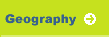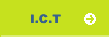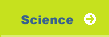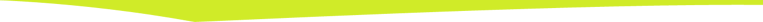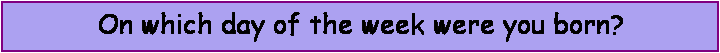This formula will work out your birth'DAY' my D.O.B. is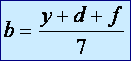1) Write down the year, in full, in which you were born y =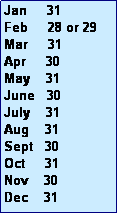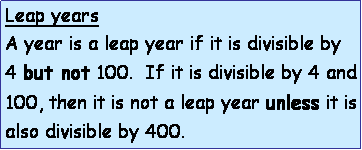2) Work out the TOTAL number of days up to and including your birthday in the year in which you were born. Was it a leap year? d =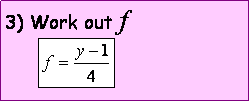f = the whole number part     only, to this answer f =
4) Work out

y + d + f
 y + d + f =
 5) Divide   (y + d + f)  by 7 and find the remainder b  is the remainder  b =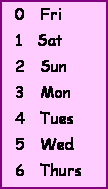6)  Convert the remainder to the day you were born, your birth'day'. I was born on a
x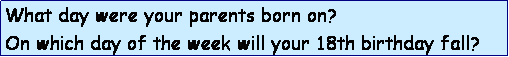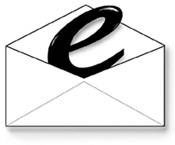Eday@huishepiscopi.somerset.sch.uk01458 250501HUISH EPISCOPI SCHOOL SCIENCE COLLEGE 2005Feedback